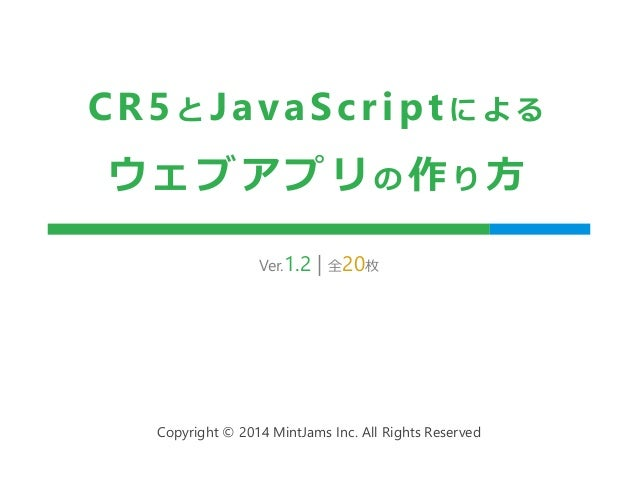Successfully reported this slideshow.Upcoming SlideShare
×

# CR5とJavaScriptによるウェブアプリの作り方

1,027 views

Published on

CR5で体験する初めてのWebアプリケーション

Published in: Technology
• Full Name
Comment goes here.

Are you sure you want to Yes NoAre you sure you want to  Yes  No

Are you sure you want to  Yes  No

Are you sure you want to  Yes  No

Are you sure you want to  Yes  No

Are you sure you want to  Yes  No
• Be the first to like this

### CR5とJavaScriptによるウェブアプリの作り方

2. 2. はじめに 対象 – ウェブブラウザで動くJavaScriptが書ける人 準備するもの – Content Repository 5.5以降 – Content Management Tools Standard 3.7以降 2 インストール方法については Getting Startedを参照してください www.slideshare.net/mintjams/getting-started-31643179
3. 3. ウェブアプリの作り方 3 Content Repository 5を活用すれば JavaScriptだけで、簡単・迅速に作れる 設計 作成 配信 実行
4. 4. 設計 4 入力された名前を表示する、 単純なウェブアプリを作る 入力 HTTP（POST）通信 名前を入力する 送信 名前を表示する 出力
5. 5. 設計 | 入力フォーム 5 名前を入力する 送信 名前を表示する  標準的なHTMLフォームにする  名前を入力するテキストボックスと 送信ボタンを配置する
6. 6. 設計 | 入力フォームの送信 6 名前を入力する 送信 名前を表示する  HTMLフォームの標準機能を使って POST送信する
7. 7. 設計 | 送信データの表示 7 名前を入力する 送信 名前を表示する  サーバ側でJavaScr iptを実行して、 HTMLに名前を出力する  サーバ側でJavaScr iptを実行する方法は 以下の2通り HTMLに直接 JavaScriptを書く テンプレートを使用して スクリプトエンジンで実行 今回はこちらを使用
8. 8. 設計 | ページ遷移図 8 入力ページ 送信 表示ページ index.html hello.html 入力された名前を表示する、 単純なウェブアプリを作る
9. 9. ウェブアプリの作り方 9 Content Repository 5を活用すれば JavaScriptだけで、簡単・迅速に作れる 設計 作成 配信 実行
10. 10. 作成 | 入力フォーム < f o rm a c t i o n = " h e l l o . h t ml " me t h o d = " p o s t " > < d i v > <input t ype=" t e x t " name="h e l lo"> < s p a n > テキストを入力して、送信ボタンを・・・< / s p a n > < / d i v > < d i v > < i n p u t t y p e = " s u bmi t " n ame = " s e n d " v a l u e = " 送信" > < i n p u t t y p e = " r e s e t " n a me = " r e s e t " v a l u e = " リセット" > < / d i v > < / f o rm> 10 名前を入力する 送信 名前を表示する index.html
11. 11. 作成 | 入力フォームの送信 名前を入力する 送信 <form a c t ion="h e l lo.h tml " me thod="pos t "> < d i v > < i n p u t t y p e = " t e x t " n a me = " h e l l o " > < s p a n > テキストを入力して、送信ボタンを・・・< / s p a n > < / d i v > < d i v > < i n p u t t y p e = " s u bmi t " n ame = " s e n d " v a l u e = " 送信" > < i n p u t t y p e = " r e s e t " n a me = " r e s e t " v a l u e = " リセット" > < / d i v > < / f o rm> 11 名前を表示する index.html
12. 12. 作成 | 送信データの表示 名前を入力する 名前を表示する < p > こんにちは、 <s c r ipt runa t="s e r v e r "> document .wr i t e ( r eque s t .ge t E s c aped ( "h e l lo" ) ) ; </ s c r ipt> さん< / p > 12 送信 hello.html
13. 13. ウェブアプリの作り方 13 Content Repository 5を活用すれば JavaScriptだけで、簡単・迅速に作れる 設計 作成 配信 実行